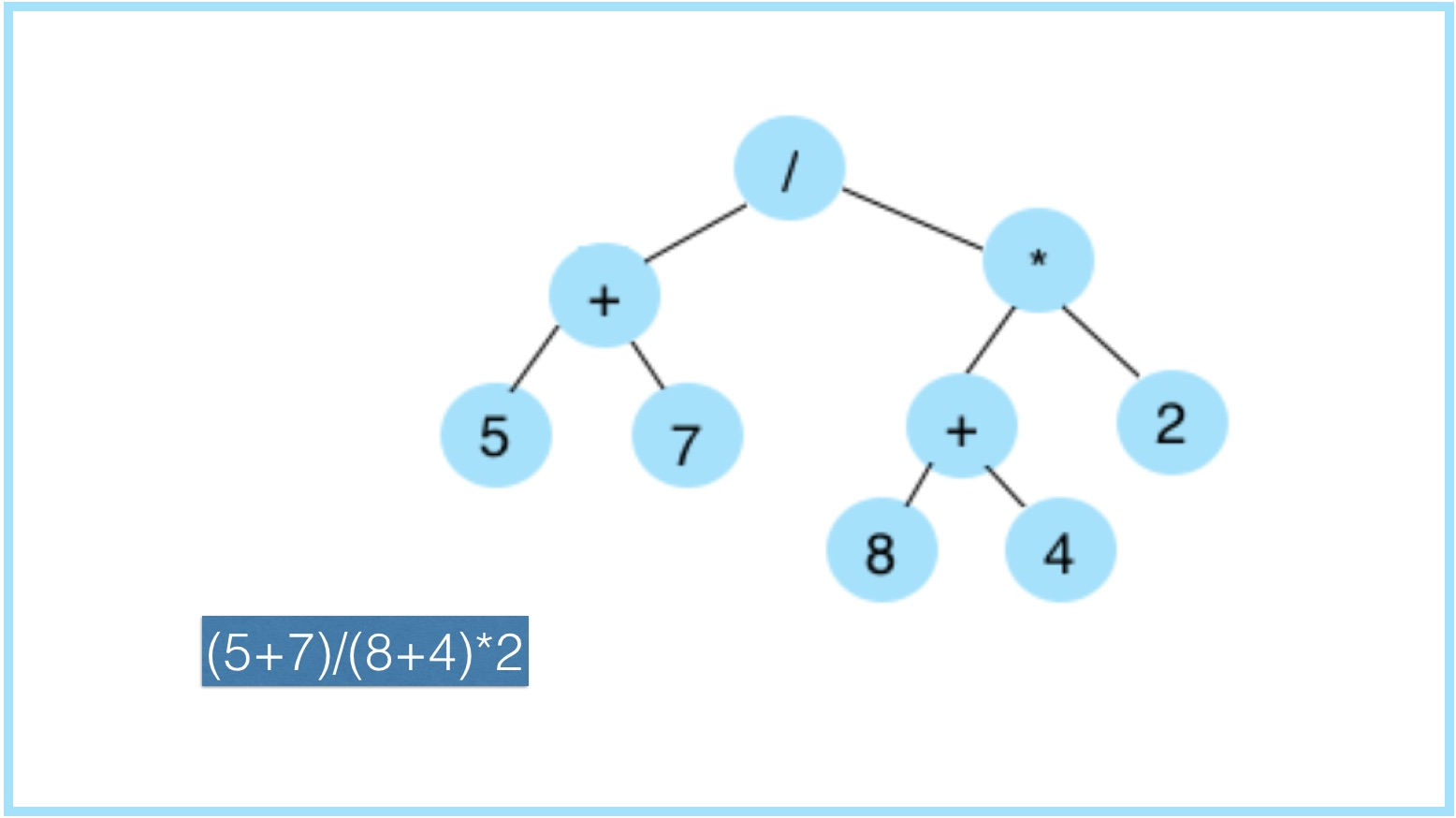## Infix expression with minimum parentheses## Infix expression with minimum parentheses

Infix, prefix and postfix are three different but equivalent ways of writing an arithmetic expressions. These expressions represent same BXT (Binary Expression Tree) while traversing the tree in different order.

### Infix Expression

Infix expression are written as we write mathematical expressions where operators are used in-between the operands e.g. a + b - c

It is easy for us humans to read, write, and speak in infix notation but it's difficult for computers to evaluate infix expression as it involves precedence and associativity of the operators. An algorithm to process infix expression could be difficult and costly in terms of time and space consumption.

### Generating Infix Expression

To generate infix expression a BXT has to be traversed Inorder which is Left-Root-Right.

Traversal will be done recursively.

#### Inorder Traversal (pseudo code):

`void inorder(root){   if (root == null){      return;   }   inorder(root.left)   print(root.data)   inorder(root.right)}`

#### Infix Traversal with minimum parentheses:

Rules to enclose the expression within parenthesis or not depends on relationship between operator and the child operator.

Expression has to be enclosed in parenthesis when:

1. operator is * and child operator is either + or -
2. operator is / and child operator is either +, - or *
`String getInfixExpr(Node node) {        if (node == null)            return "";        String expr = "";        /* first recur on left child */        expr = getInfixExpr(node.left);        /* Rule of division is different from multiplication */        if ((node.key == "*" && (node.left.key == "+" || node.left.key == "-"))                 || (node.key == "/" && (node.left.key == "+" || node.left.key == "-" || node.left.key == "*"))) {            expr = "( " + expr + ") " + node.key + " ";        } else {            expr += node.key + " ";    }            /* Rule of division is different from multiplication */    if ((node.key == "*" && (node.right.key == "+" || node.right.key == "-"))             || (node.key == "/" && (node.right.key == "+" || node.right.key == "-" || node.right.key == "*"))) {                expr += "( " + getInfixExpr(node.right) + ") ";    } else {        expr += getInfixExpr(node.right);    }    return expr;}`